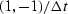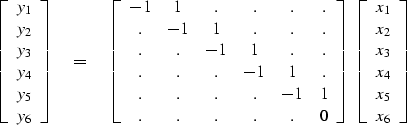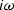Next: Zero padding is the Up: FAMILIAR OPERATORS Previous: FAMILIAR OPERATORS

Given a sampled signal, its time derivative can be estimated by convolution with the filter,expressed as the matrix-multiply below:(3)
Technically the output should be n-1 points long, but I appended a zero row, a small loss of logical purity, so that the size of the output vector will match that of the input. This is a convenience for plotting and for simplifying the assembly of other operators building on this one.

The filter impulse response is seen in any column in the middle of the matrix, namely (1,-1). In the transposed matrix, the filter-impulse response is time-reversed to (-1,1). So, mathematically, we can say that the adjoint of the time derivative operation is the negative time derivative. This corresponds also to the fact that the complex conjugate ofis.We can also speak of the adjoint of the boundary conditions: we might say that the adjoint of no boundary condition'' is a specified value'' boundary condition.

A complicated way to think about the adjoint of equation (3) is to note that it is the negative of the derivative and that something must be done about the ends. A simpler way to think about it is to apply the idea that the adjoint of a sum of N terms is a collection of N assignments. This is done in subroutine igrad1(), which implements equation (3) and its adjoint.

subroutine igrad1( adj, add,  xx,n,  yy   )
real                          xx(n), yy(n)
do i= 1, n-1 {
yy(i) = yy(i) + xx(i+1) - xx(i)
else {
xx(i+1) = xx(i+1) + yy(i)
xx(i  ) = xx(i  ) - yy(i)
}
}
return; end


Notice that the do loop in the code covers all the outputs for the operator itself, and that in the adjoint operation it gathers all the inputs. This is natural because in switching from operator to adjoint, the outputs switch to inputs.

As you look at the code, think about matrix elements being +1 or -1 and think about the forward operator pulling'' a sum into yy(i), and think about the adjoint operator pushing'' or spraying'' the impulse yy(i) back into xx().

You might notice that you can simplify the program by merging the erase output'' activity with the calculation itself. We will not do this optimization however because in many applications we do not want to include the erase output'' activity. This often happens when we build complicated operators from simpler ones.Next: Zero padding is the Up: FAMILIAR OPERATORS Previous: FAMILIAR OPERATORS
Stanford Exploration Project
12/26/2000